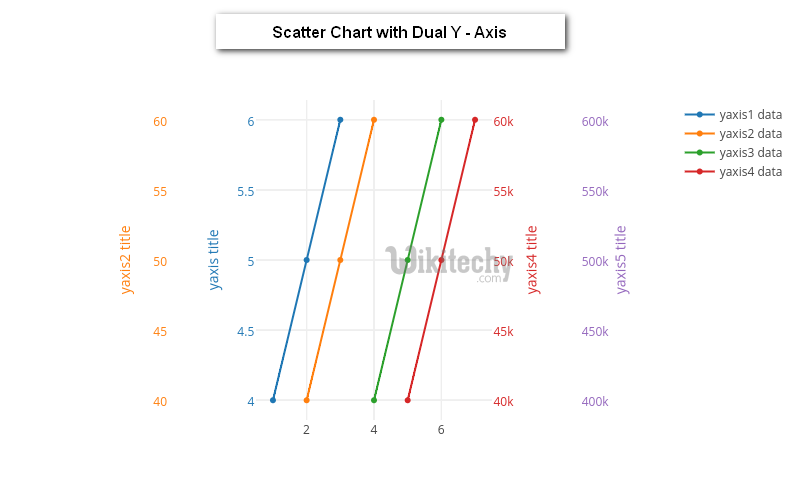# Google Charts - Google Charts tutorial - Scatter Chart with Dual Y-axis - chart js - google graphs - google charts examples

## What is Scatter Chart with Dual Y-axis?

• Scatter Charts with Dual Y- axis is a type of chart which is done by using X and Y axis coordinates to display the values for X and dual Y variables
• Scatter Charts with Dual Y- Axis are similar to line graphs which are done with X and dual Y axis where they use X and dual Y axes to plot axis points
• Scatter Charts with Dual Y- Axis show us as per how much X axis is affected by dual Y - axis and the relationship between X-axis and dual Y-Axis is called correlation.
• Scatter Charts with Dual Y- Axis are used when the continuous variable of one Y axis is controlling the experiment and the other continuous variable of Y axis depends on the controlling the experiment
• Scatter Charts with Dual Y - Axis can suggest various kinds of correlations between X - axis and dual Y - axis with a certain confidence interval.## Configuration

• The syntax which is given below gives us the configuration of Scatter Chart with Dual Y-axis and we have used axes.y configuration to show dual y axes.

## Syntax:

• The sample code which is given below show us the full sample code of scatter chart with Dual Y - Axis.